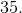# SSAT Elementary Level Math : How to find the whole from the part

## Example Questions

### Example Question #7 : Count, Read, And Write Numbers 1 120: Ccss.Math.Content.1.Nbt.A.1

What number is fifty-seven?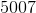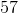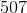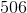Explanation:

In number form, fifty-seven is.

### Example Question #8 : Count, Read, And Write Numbers 1 120: Ccss.Math.Content.1.Nbt.A.1

What number is seventy-eight?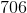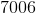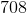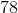Explanation:

In number form, seventy-eight is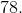### Example Question #2 : Number & Operations In Base Ten

What number is eighty-nine?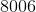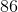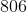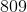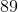Explanation:

In number form, eighty-nine is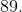### Example Question #10 : Count, Read, And Write Numbers 1 120: Ccss.Math.Content.1.Nbt.A.1

What number is one hundred eleven?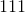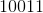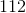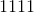Explanation:

In number form, one hundred eleven is.

### Example Question #11 : Extend The Counting Sequence

What number is one hundred seventeen?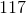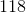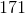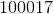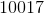Explanation:

In number form, one hundred seventeen is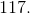### Example Question #12 : Extend The Counting Sequence

What number is twelve?Explanation:

In number form, twelve is.

### Example Question #1 : Compare Two Digit Numbers: Ccss.Math.Content.1.Nbt.B.3

Which statement is true?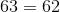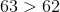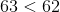Explanation:

The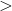sign means greater than.is greater than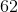because of the number in the ones place.has a greater value than, which meanshas a greater value than### Example Question #2 : Compare Two Digit Numbers: Ccss.Math.Content.1.Nbt.B.3

Which statement is true?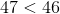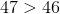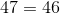Explanation:

Thesign means greater than.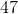is greater thanbecause of the number in the ones place.has a greater value than, which meanshas a greater value than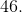### Example Question #3 : Compare Two Digit Numbers: Ccss.Math.Content.1.Nbt.B.3

Which statement is true?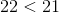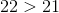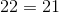Explanation:

Thesign means greater than.is greater thanbecause of the number in the ones place.has a greater value than, which meanshas a greater value than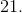### Example Question #4 : Compare Two Digit Numbers: Ccss.Math.Content.1.Nbt.B.3

Which statement is true?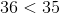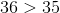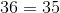Thesign means greater than.is greater thanbecause of the number in the ones place.has a greater value than, which meanshas a greater value than